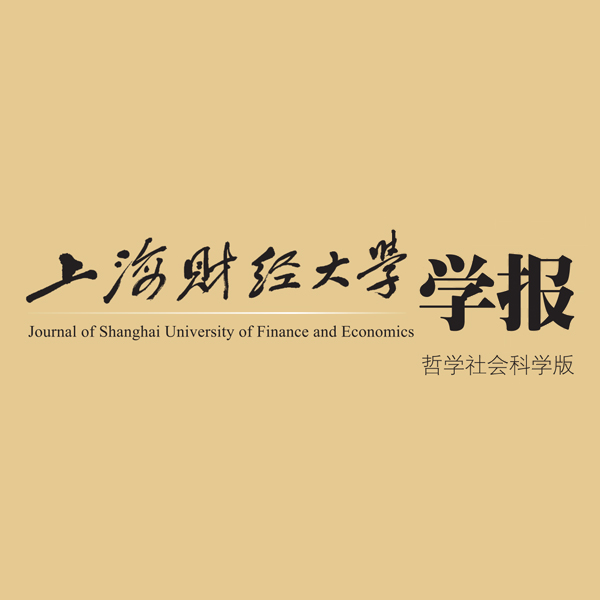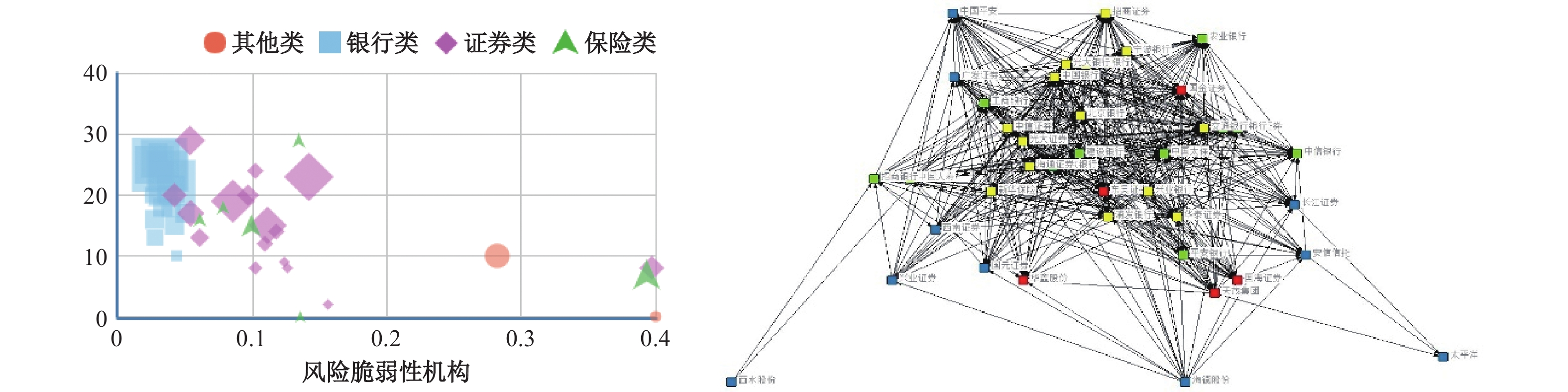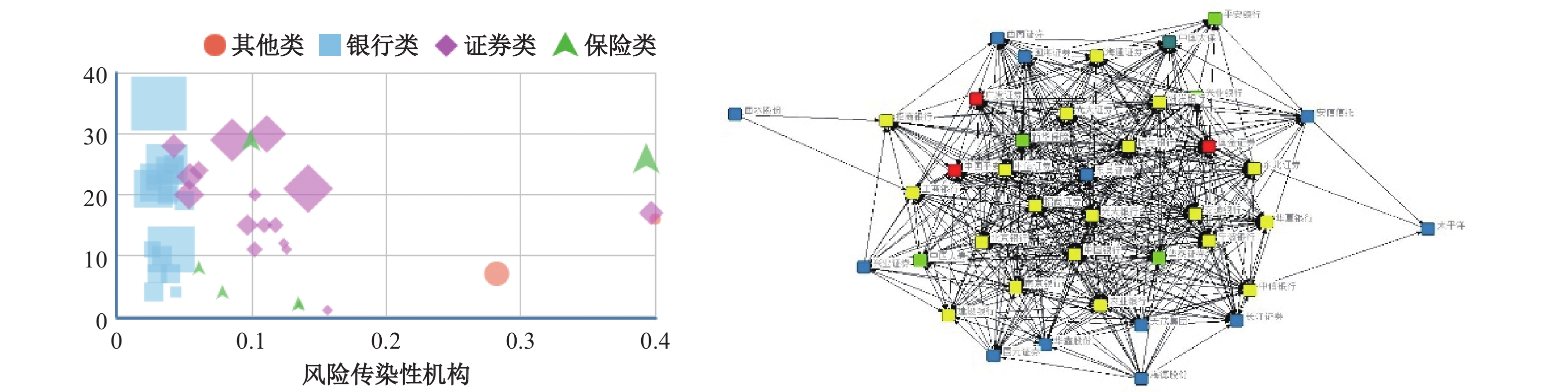﻿ 金融机构关联网络、风险溢出与系统性风险《上海财经大学学报》
2020第22卷第5期

1.吉林大学 数量经济研究中心，吉林 长春 130012;
2.吉林大学 商学院，吉林 长春 130012

2020年中央经济工作会议的重中之重是维护经济稳定。稳定目标需要防范和化解重大风险，尤其是在新冠肺炎疫情突发状况下，如何防范风险是维护金融稳定的重要保障。系统性风险的集聚和爆发离不开金融机构的参与，借助金融机构之间的关联关系，风险得以传播并产生连锁性波及。近年来，随着金融创新的逐步推进，金融机构之间的交互发展和混业经营在促进金融业发展的同时，也强化了机构间的关联性。金融机构之间形成了复杂的关联网络，这使得单一金融机构陷入困境后风险溢出会借由关联网络在系统间传播，最终导致系统性危机的爆发。2008年金融危机不仅源于金融周期波动和资产价格波动，而且来自于金融机构相互之间的密切关联。美国金融危机调查委员会调查报告指出，在金融危机期间，美国重要的13家金融机构中的12家都曾处于倒闭的边缘（FCIC,2011）。金融机构是防范系统性风险的关键所在，危机的发生通常首先影响的是金融机构，并继而向其他领域扩散。Hart和Zingales（2009）认为系统性风险是单个机构倒闭或部门崩溃，造成损失并以多米诺骨牌式的连锁反应在系统内快速传播。IMF等（2009）认为系统性风险是金融系统遭受冲击导致服务功能受损，进而对实体经济产生严重负面影响的风险。

$△\mathrm{C}\mathrm{o}\mathrm{V}\mathrm{a}\mathrm{R}$

${\mathrm{P}\mathrm{C}\mathrm{A}\mathrm{S}}_{\mathrm{i}.\mathrm{n}}$

(一) 金融机构风险溢出水平测度：
${\mathrm{P}\mathrm{C}\mathrm{A}\mathrm{S}}_{\mathrm{i}.\mathrm{n}}$

${\mathrm{P}\mathrm{C}\mathrm{A}\mathrm{S}}_{\mathrm{i}.\mathrm{n}}$

 ${PCAS}_{i.n}=\frac{1}{2}{(\sigma }_{i}^{2}/{\sigma }_{s}^{2})\times (\partial {\sigma }_{s}^{2}/\partial {\sigma }_{i}^{2})=\left({\sum }_{k=1}^{N}{(\sigma }_{i}^{2}/{\sigma }_{s}^{2}){{l}_{ik}^{2}\lambda }_{k}|h\geqslant H\right)$ (1)

$\mathrm{h}$

$\mathrm{H}$

$\mathrm{i}$

$\mathrm{i}$

(二) 金融机构关联网络矩阵：非参数格兰杰因果关系检验

$\mathrm{i}$

$\mathrm{j}$

 $j \to i = \left\{ {\begin{array}{*{20}{c}} 1\\ 0 \end{array}} \right.\;\;\;\;\begin{array}{*{20}{c}} {j{\text{是}}i{\text{的非线性格兰杰原因}}}\\ {j{\text{非}}i{\text{的非线性格兰杰原因}}} \end{array}$ (2)

1. 网络中心性度量。金融机构之间密切的业务往来将不同机构连接成错综复杂的网络，借助Freeman等（1979）的研究，将每一个金融机构看作一个节点，从关系角度给出衡量单一金融机构网络中心性的量化指标，分别为度数中心度、中介中心度。其中，度数中心度衡量的是与单一节点直接相连的其他节点个数，其方向性又分为出度与入度两方面。将度数中心度应用于金融机构关系网络可以判断单一金融机构在金融机构系统内对其他金融机构的影响力度。一家金融机构的度数中心度越高，说明与其直接相关联的金融机构越多，因此这家金融机构在网络中就越靠近中心位置，对其他金融机构的风险传染范围就越大。中介中心度是某一节点作为其他两个相关节点关联路径中介节点的个数，如果一个节点经常出现在其他节点的关联路径中，则中介中心度越大，意味着该机构在系统内越有能力促进信息向其他节点间传播。

2. 关联网络度量。根据非参数格兰杰因果关系检验，可以判断非参数格兰杰因果关联水平（degree of nonparametric Granger causality，DGC），反映的是金融机构关联关系的密切程度，其数值越大，表明金融机构间的关系越紧密。对于

$\mathrm{N}$

$\mathrm{N} (\mathrm{N}-1)$
，计算出的DGC为：

 $DGC=\frac {1}{N\left(N-1\right)} {\sum }_{i=1}^{N}{\sum }_{j\ne i}\left(j\to i\right)$ (3)

 \begin{aligned} & Out:\left(j\to s\right)=\dfrac{1}{(N-1)}{\sum }_{i\ne j}(j\to i) \\ & In:\left(s\to j\right)=\dfrac{1}{(N-1)}{\sum }_{i\ne j}(i\to j) \\ & In+Out: (j\leftrightarrow s)=\dfrac{1}{2(N-1)}{\sum }_{i\ne j}\left[\left(i\to j\right)+(j\to i)\right] \end{aligned} (4)

$\mathrm{j}$

$\mathrm{j}$

$\mathrm{s}$

$\mathrm{O}\mathrm{u}\mathrm{t}$

$\mathrm{j}$

$\mathrm{I}\mathrm{n}$

$\mathrm{j}$

$\mathrm{I}\mathrm{n}+\mathrm{O}\mathrm{u}\mathrm{t}$

 \begin{aligned} & Out-to-Other:\left(j|\alpha \right)\to {\sum }_{\beta \ne \alpha }\left(S|\beta \right)=\dfrac{1}{(M-1)N/M}{\sum }_{i\ne j}{\sum }_{\beta \ne \alpha }\left[\left(j|\alpha \right)\to \left(i|\beta \right)\right]\\ & IN-from-Other:{\sum }_{\beta \ne \alpha }\left[\left(S|\beta \right)\to \left(j|\alpha \right)\right]=\dfrac{1}{(M-1)N/M}{\sum }_{i\ne j}{\sum }_{\beta \ne \alpha }\left[\left(i|\beta \right)\to \left(j|\alpha \right)\right]\\ & IN+Out-Other:{\sum }_{\beta \ne \alpha }\left[\left(S|\beta \right)\leftrightarrow \left(j|\alpha \right)\right]=\dfrac{1}{\dfrac{2\left(M-1\right)}{N}}{\sum }_{i\ne j}{\sum }_{\beta \ne \alpha }\left[\left(i|\beta \right)\to \left(j|\alpha \right)+\left(j|\alpha \right)\to \left(i|\beta \right)\right] \end{aligned} (5)

$\mathrm{\alpha },\mathrm{\beta }$

$\mathrm{O}\mathrm{u}\mathrm{t}-\mathrm{t}\mathrm{o}-\mathrm{o}\mathrm{t}\mathrm{h}\mathrm{e}\mathrm{r}$

$\mathrm{j}$

$\mathrm{i}$

$\mathrm{I}\mathrm{n}-\mathrm{f}\mathrm{r}\mathrm{o}\mathrm{m}-\mathrm{o}\mathrm{t}\mathrm{h}\mathrm{e}\mathrm{r}$

$\mathrm{j}$

$\mathrm{i}$

$\mathrm{I}\mathrm{n}+\mathrm{O}\mathrm{u}\mathrm{t}-\mathrm{o}\mathrm{t}\mathrm{h}\mathrm{e}\mathrm{r}$

(三) 风险脆弱类与风险传染类的划分

${\mathrm{P}\mathrm{C}\mathrm{A}\mathrm{S}}_{i,n}$

${\mathrm{P}\mathrm{C}\mathrm{A}\mathrm{S}}_{i,n}$

 脆弱性划分 深度 入度 第一区（入度较高、深度较低） 第二区（入度较高、深度较高） 第三区（入度较低、深度较低） 第四区（入度较低、深度较高） 传染性划分 深度 出度 第一区（出度较高、深度较低） 第二区（出度较高、深度较高） 第三区（出度较低、深度较低） 第四区（出度较低、深度较高）

$\mathrm{R}=\mathrm{l}\mathrm{n}\left({\rm{P}}_{t}/{\rm{P}}_{t-1}\right)$
，其中
${\mathrm{P}}_{t}$

${\mathrm{P}}_{t-1}$

 部门 观测值 均值 标准差. 最小值 最大值 偏度 峰度 银行类 5520 0.001 0.044 −0.629 0.289 −1.008 22.119 证券类 5520 −7.00E−05 0.07 −0.863 0.919 −0.845 30.651 保险类 2070 0.002 0.061 −0.882 0.477 −1.08 29.865 其他类 690 −0.001 0.086 −0.975 0.440 −3.067 42.058

${\mathrm{P}\mathrm{C}\mathrm{A}\mathrm{S}}_{\mathrm{i},\mathrm{n}}$

(二) 金融机构风险溢出水平

 排名 机构 ${\mathrm{P}\mathrm{C}\mathrm{A}\mathrm{S}}_{\mathrm{i},\mathrm{n}}$ 排名 机构 ${\mathrm{P}\mathrm{C}\mathrm{A}\mathrm{S}}_{\mathrm{i},\mathrm{n}}$ 1 海德股份 0.3997 21 华泰证券 0.0607 2 华鑫股份 0.3968 22 海通证券 0.0542 3 天茂集团 0.3930 23 招商证券 0.0535 4 安信信托 0.2819 24 南京银行 0.0500 5 太平洋证券 0.1562 25 平安银行 0.0437 6 国金证券 0.1419 26 兴业银行 0.0421 7 西水股份 0.1357 27 中信证券 0.0420 8 中国平安 0.1344 28 工商银行 0.0402 9 兴业证券 0.1260 29 建设银行 0.0397 10 国元证券 0.1237 30 北京银行 0.0392 11 长江证券 0.1177 31 交通银行 0.0373 12 东吴证券 0.1112 32 中国银行 0.0369 13 西南证券 0.1093 33 中信银行 0.0334 14 国海证券 0.1022 34 宁波银行 0.0315 15 广发证券 0.1021 35 光大银行 0.0310 16 新华保险 0.0993 36 农业银行 0.0299 17 东北证券 0.0968 37 浦发银行 0.0276 18 光大证券 0.0854 38 招商银行 0.0272 19 中国太保 0.0782 39 民生银行 0.0269 20 中国人寿 0.0608 40 华夏银行 0.0261

${\mathrm{P}\mathrm{C}\mathrm{A}\mathrm{S}}_{i,n}$

${\mathrm{P}\mathrm{C}\mathrm{A}\mathrm{S}}_{i,n}$

${\mathrm{P}\mathrm{C}\mathrm{A}\mathrm{S}}_{\mathrm{i},\mathrm{n}}$

 类别 均值 最小值 最大值 银行 0.0352 0.0261 0.0500 证券 0.1175 0.0420 0.3968 保险 0.1502 0.0608 0.3930 其他 0.3408 0.2819 0.3997

(三) 金融机构关联结构

 整体 653(41.86%） 类型 DGC 简化矩阵 From/TO 银行 证券 保险 其他 银行 证券 保险 其他 银行 103（42.92%） 124（48.44%） 33（34.38%） 7（21.88%） 1 1 0 0 证券 168（65.63%） 78（32.50%） 45（46.88%） 1（3.13%） 1 0 1 0 保险 38（39.58%） 26（27.08%） 6（20.00%） 1（8.33%） 0 0 0 0 其他 8（25%） 13（40.63%） 1（8.33%） 1（50%） 0 0 0 1

 IN OUT IN+OUT IN-FROM-OTHER OUT-TO-OTHER IN+OUT-OTHER 银行 19.81 16.69 18.25 13.38 10.25 11.81 证券 15.06 18.25 16.66 7.75 13.38 10.56 保险 14.17 11.83 13.00 13.17 10.83 12.00 其他 5.00 11.50 8.25 4.50 11.00 7.75

$\mathrm{I}\mathrm{N}$

$\mathrm{O}\mathrm{U}\mathrm{T}$

(四) 金融机构风险关联网络

${\mathrm{P}\mathrm{C}\mathrm{A}\mathrm{S}}_{i,n}$

${\mathrm{P}\mathrm{C}\mathrm{A}\mathrm{S}}_{i,n}$

${\mathrm{P}\mathrm{C}\mathrm{A}\mathrm{S}}_{i,n}$

${\mathrm{P}\mathrm{C}\mathrm{A}\mathrm{S}}_{i,n}$

${\mathrm{P}\mathrm{C}\mathrm{A}\mathrm{S}}_{i,n}$

${\mathrm{P}\mathrm{C}\mathrm{A}\mathrm{S}}_{i,n}$图 1 金融机构风险脆弱性关联图图 2 金融机构风险传染性关联图

${\mathrm{P}\mathrm{C}\mathrm{A}\mathrm{S}}_{i,n}$
，Y轴为金融机构的入度/出度值，以
${\mathrm{P}\mathrm{C}\mathrm{A}\mathrm{S}}_{i,n}$
、入度/出度值均值为界将金融机构划分为四个区域。金融机构脆弱性的划分如表7所示。具体来看，第一区由20家金融机构构成，占全部样本机构数量的50%，其中包括了大部分商业银行和证券机构，关联网络共415条，其中来自其他区域影响的关联数量达204条，区域内部互相关联数量为211条，说明该区域金融机构无论是对内还是对外都具有较强的影响能力。第二区包括2家证券公司和1家保险机构，共接收关联网络76条，其中接收跨区域关联数量达72条，平均每家机构受24家其他金融机构的影响。第三区由5家机构组成，包含了2家商业银行、1家证券机构及2家保险机构，共接收69条关联网络，其中来自其他区域的关联关系59条，区域内关联关系10条。第四区包含8家证券机构、2家保险机构和全部其他类金融机构，共接收关联关系93条，其中跨区关联关系达76条，可见第四区的金融机构易受其他区域金融机构的影响。

 深度 入度 第一区 第二区 东北证券 光大证券 中国太保 海通证券 国金证券 中国平安 广发证券 招商证券 南京银行 中信证券 工商银行 建设银行 北京银行 交通银行 中国银行 中信银行 宁波银行 光大银行 农业银行 浦发银行 招商银行 民生银行 华夏银行 第三区 第四区 新华保险 中国人寿 华泰证券 平安银行 海德股份 华鑫股份 天茂集团 安信信托 兴业银行 太平洋证券 西水股份 兴业证券 国元证券 长江证券 东吴证券 西南证券 国海证券

 深度 出度 第一区 第二区 新华保险 光大证券 华泰证券 海通证券 华鑫股份 天茂集团 国金证券 东吴证券 招商证券 南京银行 兴业银行 中信证券 国海证券 北京银行 交通银行 中国银行 宁波银行 光大银行 浦发银行 民生银行 第三区 第四区 东北证券 中国太保 中国人寿 平安银行 海德股份 安信信托 太平洋证券 西水股份 工商银行 建设银行 中信银行 农业银行 中国平安 兴业证券 国元证券 长江证券 招商银行 华夏银行 西南证券 广发证券

  巴曙松, 朱虹. 金融监管模式的演进[J].中国金融,2018(7).  陈守东, 王妍. 我国金融机构的系统性金融风险评估——基于极端分位数回归技术的风险度量[J].中国管理科学,2014(7).  范小云, 王道平, 方意. 我国金融机构的系统性风险贡献测度与监管——基于边际风险贡献与杠杆率的研究[J].南开经济研究,2011(4).  蒋海, 张锦意. 商业银行尾部风险网络关联性与系统性风险——基于中国上市银行的实证检验[J].财贸经济,2018(8).  李政, 梁琪, 方意. 中国金融部门间系统性风险溢出的监测预警研究——基于下行和上行ΔCoES指标的实现与优化[J].金融研究,2019(2).  李政, 刘淇, 梁琪. 基于经济金融关联网络的中国系统性风险防范研究[J].统计研究,2019(2).  林达, 李勇. 中国上市金融机构关联性度量及影响因素分析[J].统计研究,2019(4).  欧阳红兵, 刘晓东. 中国金融机构的系统重要性及系统性风险传染机制分析——基于复杂网络的视角[J].中国管理科学,2015(10).  隋聪, 谭照林, 王宗尧. 基于网络视角的银行业系统性风险度量方法[J].中国管理科学,2016(5).  肖璞, 刘轶, 杨苏梅. 相互关联性、风险溢出与系统重要性银行识别[J].金融研究,2012(12).  杨子晖, 周颖刚. 全球系统性金融风险溢出与外部冲击[J].中国社会科学,2018(12).  章和杰, 施楚凡, 金辉, 等. 系统性金融风险测度与预警模型——一个研究综述[J].现代管理科学,2019(9).  Acharya V V, Pedersen L H, Philippon T, et al. Measuring systemic risk[J].The Review of Financial Studies,2017,30(1):2–47.  Adrian T, Brunnermeier M K. CoVaR[J].American Economic Review,2016,106(7):1705–1741.  Allen F, Gale F. Bubbles and crises[J].The Economic Journal,2000,110(460):236–255.  Billio M, Getmansky M, Lo A W, et al. Econometric measures of connectedness and systemic risk in the finance and insurance sectors[J]. Journal of Financial Economics,2017,104(3):535–559.  Brownlees C, Engle R F. SRISK: A conditional capital shortfall measure of systemic risk[J]. The Review of Financial Studies,2016,30(1):48–79.  Chen C Y H, Härdle W K, Okhrin Y. Tail event driven networks of SIFIs[J].Journal of Econometrics,2019,208(1):282–298.  Diamond D, Dybvig P. Bank runs, deposit insurance, and liquidity[J].Journal of Political Economy,1983,91(3):401–419.  Diebold F X, Yılmaz K. On the network topology of variance decompositions: Measuring the connectedness of financial firms[J].Journal of Econometrics,2014,182(1):119–134.  Diks C, Panchenko V. A new statistic and practical guidelines for nonparametric Granger causality testing[J].Journal of Economic Dynamics and Control,2006,30(9-10):1647–1669.  Fisher I. The debt-deflation theory of great depressions[J].Econometrica,1933,1(4):337–357.  Freeman L C, Roeder D, Mulholland R R. Centrality in social networks: Ⅱ. experimental results[J].Social Networks,1979,2(2):119–141.  Geraci M V, Gnabo J Y. Measuring interconnectedness between financial institutions with Bayesian Time－Varying vector autoregressions[J].Journal of Financial and Quantitative Analysis,2018,53(3):1371–1390.  Hart O, Zingales L. How to avoid a new financial crisis[R]. University of Chicago Booth School, Business Research Paper, 2009.  IMF, BIS, FSB. Guidance to assess the systemic importance of financial institutions[R]. Markets and Instruments: Initial Considerations, 2009.  Kupiec P, Nickerson D. Assessing systemic risk exposure from banks and GSEs under alternative approaches to capital regulation[J]. Journal of Real Estate Finance and Economics,2004,28(2):123–145.  Minsky H P. The financial instability hypothesis[R]. The Jerome Levy Economics Institute, Working Paper No. 74, 1992.  Molyneux P, Schaeck K, Zhou T M. ‘Too systemically important to fail’ in banking – Evidence from bank mergers and acquisitions[J].Journal of International Money and Finance,2014,49:258–282.  Papana A, Kyrtsou C, Kugiumtzis D. Financial networks based on Granger causality: A case study[J]. Physica A: Statistical Mechanics and its Applications,2017(482):65–73.  Skripnikov A, Michailidis G. Joint estimation of multiple network Granger causal models[J]. Econometrics and Statistics,2019(10):120–133.  Sullivan H, Yannick L, Sessi T. Measuring network systemic risk contributions: A leave-one out approach[J].Journal of Economic Dynamic and Control,2019,100:86–114.
Incidence Network, Risk Spillover and Systemic Risk of Financial Institutions
Zhang Ailian1, Zhang Yang2
1.Center for Quantitative Economics, Jilin University, Jilin Changchun 130012, China;
2.Business School, Jilin University, Jilin Changchun 130012, China
Abstract: Systemic risk is closely related to the risk contagion of financial institutions. The risk of a single financial institution will spread among financial systems through the complex incidence network among financial institutions, and lead to the outbreak of crisis. However, the risk contagion paths of different financial institutions are different, and the degree of impact is significantly different. The research of using the reduced-form approach mostly focuses on measuring the risk level of a single institution in the system, but there is relatively little literature on the measurement of risk spillover level among financial institutions and different types of financial sectors. The existing research for complex network model analysis is still focused on the construction of multiple symmetric correlation matrix, and the analysis of financial institutions through the construction of asymmetric correlation matrix is still a small number. Based on the classification of commercial banks, securities companies, insurance institutions and other types of financial institutions, this paper measures the impact of specific financial institutions on systemic risk from the perspective of risk spillover degree of financial institutions. Then, according to the nonparametric Granger causality test, the incidence matrix of financial institutions is constructed, and the financial institutions are used as nodes to depict and describe the incidence network indicators and network center properties of financial institutions. At the same time, the risk area is divided by combining the risk spillover index and the input/output value of financial institutions, so as to clearly identify the vulnerability of risk tolerance and the infectivity of risk transmission of financial institutions, in order to judge the risk prevention core and the key of targeted measures. The empirical results show that banking financial institutions are the core of China’s financial incidence network, and they have high stability. Securities financial institutions are at a high level in the level of systemic risk spillover and the degree of financial network connection, and they are the key sectors for China’s systemic risk prevention. The vulnerability and infectivity of different financial institutions are different, and the vulnerability and infectivity of the same financial institution are also different. The increase of capital scale does not mean the increase of risk contagion and vulnerability. Therefore, the risk identification of financial institutions should be comprehensive, not limited to a single standard. On the basis of focusing on the scale of financial institutions, combined with the direction, path and degree of risk impact of specific financial institutions, the risk prevention core is determined. At the same time, according to the different characteristics of risk vulnerability and risk contagion of financial institutions, we should implement targeted regulatory measures.
Key words: financial institutions    systemic risk    incidence network    risk spillover